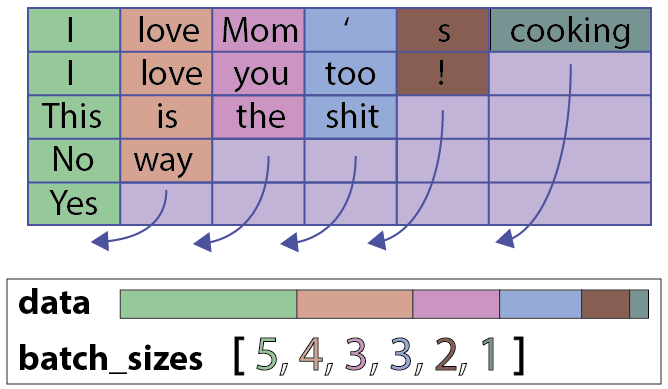# pytorch对可变长度序列的处理

• 时间:

`主要是用函数torch.nn.utils.rnn.``PackedSequence()和torch.nn.utils.rnn.pack_padded_sequence()以及torch.nn.utils.rnn.pad_packed_sequence()来进行的,分别来看看这三个函数的用法。`

1、`torch.nn.utils.rnn.``PackedSequence()`

`NOTE：` 这个类的实例不能手动创建。它们只能被 `pack_padded_sequence()` 实例化。

PackedSequence对象包括：

• 一个`data`对象：一个torch.Variable（令牌的总数，每个令牌的维度），在这个简单的例子中有五个令牌序列（用整数表示）：（18，1）
• 一个`batch_sizes`对象：每个时间步长的令牌数列表，在这个例子中为：[6，5，2，4，1]PackedSequence对象有一个很不错的特性，就是我们无需对序列解包（这一步操作非常慢）即可直接在PackedSequence数据变量上执行许多操作。特别是我们可以对令牌执行任何操作（即对令牌的顺序/上下文不敏感）。当然，我们也可以使用接受PackedSequence作为输入的任何一个pyTorch模块（pyTorch 0.2）。

2、`torch.nn.utils.rnn.pack_padded_sequence()`

`Variable`中保存的序列，应该按序列长度的长短排序，长的在前，短的在后。即`input[:,0]`代表的是最长的序列，`input[:, B-1]`保存的是最短的序列。

`NOTE：` 只要是维度大于等于2的`input`都可以作为这个函数的参数。你可以用它来打包`labels`，然后用`RNN`的输出和打包后的`labels`来计算`loss`。通过`PackedSequence`对象的`.data`属性可以获取 `Variable`

• input (Variable) – 变长序列 被填充后的 batch

• lengths (list[int]) – `Variable` 中 每个序列的长度。

• batch_first (bool, optional) – 如果是`True`，input的形状应该是`B*T*size`

3、`torch.nn.utils.rnn.pad_packed_sequence()`

Batch中的元素将会以它们长度的逆序排列。

• sequence (PackedSequence) – 将要被填充的 batch

• batch_first (bool, optional) – 如果为True，返回的数据的格式为 `B×T×*`

 1 2 3 4 5 6 7 8 9 10 11 12 13 14 15 16 17 18 19 20 21 22 23 24 25 26 `import torch` `import torch.nn ``as` `nn` `from` `torch.autograd import Variable` `from` `torch.nn import utils ``as` `nn_utils` `batch_size = 2` `max_length = 3` `hidden_size = 2` `n_layers =1`   `tensor_in = torch.FloatTensor([[1, 2, 3], [1, 0, 0]]).resize_(2,3,1)` `tensor_in = Variable( tensor_in ) #[batch, seq, feature], [2, 3, 1]` `seq_lengths = [3,1] # list of integers holding information about the batch size at each sequence step`   `# pack it` `pack = nn_utils.rnn.pack_padded_sequence(tensor_in, seq_lengths, batch_first=True)`   `# initialize` `rnn = nn.RNN(1, hidden_size, n_layers, batch_first=True)` `h0 = Variable(torch.randn(n_layers, batch_size, hidden_size))`   `#forward` `out``, _ = rnn(pack, h0)`   `# unpack` `unpacked = nn_utils.rnn.pad_packed_sequence(``out``)` `print(``'111'``,unpacked)`

输出：

 1 2 3 4 5 6 7 8 9 10 `111 (Variable containing:` `(0 ,.,.) =` `  ``0.5406  0.3584` ` ``-0.1403  0.0308`   `(1 ,.,.) =` ` ``-0.6855 -0.9307` `  ``0.0000  0.0000` `[torch.FloatTensor of size 2x2x2]` `, [2, 1])`